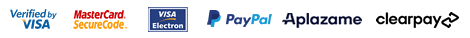▼ Language: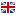English

##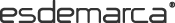WOMAN | MAN | KIDS
Favourites
My account# Buy DIM Boxers for Man

Brands
Sizes

Select
Price

Select
Colour
Select
Style

Select
Material

Select
 Order Relevance Ascending price Descending price Discount New in Delivery date Ratings SHOES10 -10% EXTRA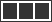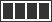### DIM Pack of 2 Unno by DIM Basic boxers, light blue, marine

14.30 €
 Size 3 = 48 4 = 52 5 = 56 6 = 60 Add
Sizes: 3 = 48   4 = 52   5 = 56   6 = 60

### DIM Pack of 2 boxers red, blue

28.90 €
 Size 3/M 4/L 5/XL 6/XXL Add
Sizes: 3/M   4/L   5/XL   6/XXL

### DIM Pack of 3 basic boxer shorts black, brown, blue

30.90 €
 Size 3/M 4/L 5/XL 6/XXL Add
Sizes: 3/M   4/L   5/XL   6/XXL

31.90 €
Sizes: 3 = 48

### DIM Pack of 2 boxers blue, grey

28.90 €
 Size 3/M 4/L 5/XL 6/XXL Add
Sizes: 3/M   4/L   5/XL   6/XXL

### DIM Pack of 3 basic multicolor boxers

30.90 €
 Size 3 = 48 4 = 52 5 = 56 6 = 60 Add
Sizes: 3 = 48   4 = 52   5 = 56   6 = 60

### DIM Pack of 3 basic boxer shorts brown-black, blue, brown

30.90 €
 Size 3/M 4/L 5/XL 6/XXL Add
Sizes: 3/M   4/L   5/XL   6/XXL

### DIM Pack of 2 multicoloured boxers

9.99 €
 Size 3 = 48 4 = 52 5 = 56 6 = 60 Add
Sizes: 3 = 48   4 = 52   5 = 56   6 = 60

### DIM Pack of 2 blue seamless boxers

14.30 €
 Size 3/M 4/L 5/XL 6/XXL Add
Sizes: 3/M   4/L   5/XL   6/XXL

### DIM Pack of 2 boxers blue, white

28.90 €
 Size 3/M 4/L 5/XL 6/XXL Add
Sizes: 3/M   4/L   5/XL   6/XXL

### DIM Pack of 2 boxers seamless blue, black

14.30 €
 Size 3/M 4/L 5/XL 6/XXL Add
Sizes: 3/M   4/L   5/XL   6/XXL

### DIM Pack of 2 seamless boxer shorts multicolor

31.90 €
 Size 3 = 48 4 = 52 Add
Sizes: 3 = 48   4 = 52

### DIM Pack of 2 Cotton Stretch Boxers Basic and Economic Style red, gray

19.90 €
 Size 2 = 44 3 = 48 4 = 52 5 = 56 Add
Sizes: 2 = 44   3 = 48   4 = 52   5 = 56

### DIM Pack of 2 Cotton Stretch Boxers Basic and Economical Style blue

19.90 €
 Size 3 = 48 4 = 52 5 = 56 Add
Sizes: 3 = 48   4 = 52   5 = 56

### DIM Pack of 2 Unno by DIM Basic boxers, grey, purple

14.30 €
 Size 3 = 48 4 = 52 5 = 56 6 = 60 Add
Sizes: 3 = 48   4 = 52   5 = 56   6 = 60

### DIM Pack of 3 blue, light blue, black elastic cotton boxers

39.90 €
 Size 2 = 44 3 = 48 4 = 52 5 = 56 Add
Sizes: 2 = 44   3 = 48   4 = 52   5 = 56

### DIM Pack of 3 light grey, dark grey, black cotton boxers

35.00 €
 Size 3 = 48 4 = 52 6 = 60 Add
Sizes: 3 = 48   4 = 52   6 = 60

### DIM Pack of 2 black cotton boxers with coloured waistband

23.90 €
 Size 2 = 44 4 = 52 Add
Sizes: 2 = 44   4 = 52

### DIM Pack of 2 Unno by DIM Basic boxers white, black

14.30 €
 Size 3 = 48 4 = 52 5 = 56 6 = 60 Add
Sizes: 3 = 48   4 = 52   5 = 56   6 = 60

### DIM Pack of 2 cotton boxer shorts with striking blue print waistbands

20.90 €
 Size 2 = 44 3 = 48 4 = 52 5 = 56 Add
Sizes: 2 = 44   3 = 48   4 = 52   5 = 56

### DIM Pack of 2 sport boxers, ultra elastic fabric, blue

31.90 €
 Size 3 = 48 4 = 52 5 = 56 Add
Sizes: 3 = 48   4 = 52   5 = 56

### DIM Pack of 2 long sports microfibre boxers with breathable mesh grey, black

29.90 €
Sizes: 2   3   4

### DIM 2-pack of blue, grey motion-adjustable boxer shorts

31.90 €
 Size 2 = 44 3 = 48 4 = 52 5 = 56 6 = 60 Add
Sizes: 2 = 44   3 = 48   4 = 52   5 = 56   6 = 60

### DIM Pack of 2 Unno by DIM Basic boxers, burgundy, black

14.30 €
 Size 3 = 48 4 = 52 5 = 56 6 = 60 Add
Sizes: 3 = 48   4 = 52   5 = 56   6 = 60

### DIM Pack of 2 cotton boxer shorts with striking grey printed waistbands

20.90 €
 Size 2 = 44 3 = 48 4 = 52 5 = 56 Add
Sizes: 2 = 44   3 = 48   4 = 52   5 = 56

### DIM Pack of 2 Unno by DIM Basic black boxers

14.30 €
 Size 2 = 44 3 = 48 4 = 52 5 = 56 6 = 60 Add
Sizes: 2 = 44   3 = 48   4 = 52   5 = 56   6 = 60

### DIM Pack of 3 light blue, navy, blue cotton boxers

35.00 €
 Size 5 = 56 6 = 60 Add
Sizes: 5 = 56   6 = 60

### DIM Pack of 2 Unno by DIM Basic boxers blue, grey

14.30 €
 Size 3 = 48 4 = 52 5 = 56 6 = 60 Add
Sizes: 3 = 48   4 = 52   5 = 56   6 = 60

### DIM Pack of 2 black elastic cotton boxers

35.00 €
 Size 2 = 44 3 = 48 4 = 52 5 = 56 Add
Sizes: 2 = 44   3 = 48   4 = 52   5 = 56

### DIM Pack of 2 Unno by DIM Basic grey, black

14.30 €
 Size 3 = 48 4 = 52 5 = 56 6 = 60 Add
Sizes: 3 = 48   4 = 52   5 = 56   6 = 60

### DIM Pack of 2 black elastic cotton boxers

31.90 €
 Size 2 = 44 3 = 48 4 = 52 5 = 56 6 = 60 Add
Sizes: 2 = 44   3 = 48   4 = 52   5 = 56   6 = 60

### DIM Pack of 2 Unno by DIM Basic boxers black, fuchsia

14.30 €
 Size 3 = 48 4 = 52 5 = 56 6 = 60 Add
Sizes: 3 = 48   4 = 52   5 = 56   6 = 60

### DIM Pack of 3 black elastic cotton boxers

30.90 €
 Size 3 = 48 4 = 52 5 = 56 6 = 60 Add
Sizes: 3 = 48   4 = 52   5 = 56   6 = 60

### DIM Pack of 2 Unno by DIM Basic black boxers

10.40 €
13.00 €
 Size 5 = 56 3 = 48 4 = 52 6 = 60 Add
Sizes: 5 = 56   3 = 48   4 = 52   6 = 60

### DIM Pack of 3 black, grey, white elastic cotton boxers

23.92 €
29.90 €
 Size 3 = 48 4 = 52 5 = 56 6 = 60 2 = 44 Add
Sizes: 3 = 48   4 = 52   5 = 56   6 = 60   2 = 44
 Order Relevance Ascending price Descending price Discount New in Delivery date Ratings SHOES10 -10% EXTRA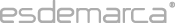Esdemarca Esdemarca Our commitments Privacy policy About cookies Your orders Help Security Shipping costs Easy return Contacts Are you a supplier? Join our team Customer service Make your suggestion Connect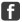Facebook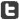Twitter Go to mobile versionEsdemarca · Copyright © Fusion Lab, S.L. 2021  |  Legal warning B66138827 · Calle Manzanares, 4, 28005, Madrid (España) · consultas@esdemarca.com · +34 91 769 72 17